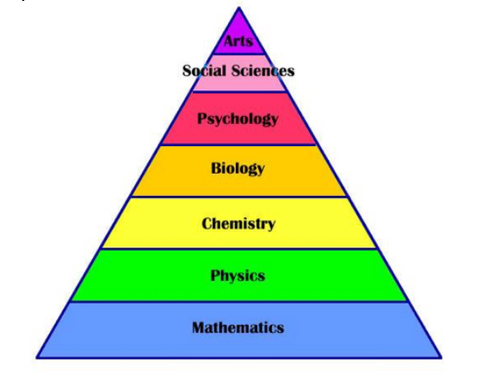# Key Concepts Required For GAMSAT: The Physical Sciences And Chemical Systems

The physical sciences lie at the heart of all the other disciplines relevant to Section III of GAMSAT. This is reflected in the hierarchy of sciences:The key implication of the hierarchy is that all sciences are premised on irreducible physical laws (mathematics). Even the biological and physiological processes witnessed on a macroscopic level are at their core explainable by these physical laws. The division of sciences in GAMSAT is 40% biology (including physiology), 40% chemistry (20 % organic and 20% physical), and 20% mathematical physics. Hence, 40% of the Section III exam is comprised of what would be classified as “hard” or classical sciences in the form of physical chemistry, physics, and mathematics. The other sciences are more applied. What this means is that the higher-level sciences describe concepts not based on their own laws or general principles. Subjects such as biology and physiology (and medicine) are somewhat arbitrary in that their level of focus is not premised on universal laws. This makes them less efficient to study, but in some instances more interesting.

The “softer” sciences are, however, premised in the universal laws of physics (ergo mathematics). This is an important consideration for those preparing for GAMSAT as there is significant yield in comprehensive study of the physical sciences. Consider the following key broad physical concepts:

1. Energy and heat
2. Temperature
3. Matter and mass
4. Pressure
5. Volume
6. Force
7. Charge

The above represent the most important concepts in physics and physical chemistry, but also in organic chemistry, and physiology. The principles underpinning these concepts are also responsible for the biological processes many candidates’ study for GAMSAT. Understanding the above seven physical concepts is by far the highest-yield approach to studying Section III. It is these concepts that students should always seek to relate their understanding of scientific systems.

Chemical science is more proximal to these physical laws than are the human sciences; for instance, consider the concept of electrostatics. By learning the principles that underlie electrostatics and atomic anatomy, much of the organic chemistry curriculum can be surmised without the need for rote learning. It follows therefore that an understanding of electrostatics is essential for the GAMSAT. Fundamental to an understanding of chemical systems is comprehension of energy transfers during chemical reactions. Before considering energy transfer, principles students need to understand intuitively are that of the system, its surroundings, and the universe (comprising both system and surroundings). In the context of chemical systems, temperature is more superficially described as a measure of the random motion of particles in a system (or the average kinetic energy) and heat is the transfer of energy due to establishing a temperature difference.

Those who are familiar with the above will collectively recognise the concepts as the basis of the subject of chemical thermodynamics. Why is this subject important? By its understanding, students can predict the way chemical reactions will propagate. Rather than rote memorising the negative and positive signs of Gibbs Free Energy, and the spontaneity of reactions, it is more important to understand why it is so.